## EX 11.5 QUESTION 1.

State which of the following are equations (with a variable). Give reason for your answer. Identify the variable from the equations with a variable.(a) 17 = x + 17(b) (t – 7) > 5(c) 4 / 2 = 2

(d) (7 × 3) – 19 = 8

(e) 5 × 4 – 8 = 2x

(f) x – 2 = 0

(g) 2m < 30

(h) 2n + 1 = 11

(i) 7 = (11 × 5) – (12 × 4)

(j) 7 = (11 × 2) + p

(k) 20 = 5y

(l) 3q/ 2 < 5

(m) z + 12 > 24

(n) 20 – (10 – 5) = 3 × 5

(o) 7 – x = 5

Solution:

(a) An equation with variable x

(b) An inequality equation

(c) No, it’s a numerical equation

(d) No, it’s a numerical equation

(e) An equation with variable x

(f) An equation with variable x

(g) An inequality equation

(h) An equation with variable n

(i) No, it’s a numerical equation

(j) An equation with variable p

(k) An equation with variable y

(l) An inequality equation

(m) An inequality equation

(n) No, it’s a numerical equation

(o) An equation with variable x

## EX 11.5 QUESTION 2.

Complete the entries in the third column of the table.

 S.No Equation Value of variable Equation satisfiedYes / No (a) 10y = 80 y = 10 (b) 10y = 80 y = 8 (c) 10y = 80 y = 5 (d) 4l = 20 l = 20 (e) 4l = 20 l = 80 (f) 4l = 20 l = 5 (g) b + 5 = 9 b = 5 (h) b + 5 = 9 b = 9 (i) b + 5 = 9 b = 4 (j) h – 8 = 5 h = 13 (k) h – 8 = 5 h = 8 (l) h – 8 = 5 h = 0 (m) p + 3 = 1 p = 3 (n) p + 3 = 1 p = 1 (o) p + 3 = 1 p = 0 (p) p + 3 = 1 p = -1 (q) p + 3 = 1 p = -2

Solutions:

 S.No Equation Value of variable Equation satisfiedYes / No (a) 10y = 80 y = 10 NO (b) 10y = 80 y = 8 YES (c) 10y = 80 y = 5 NO (d) 4l = 20 l = 20 NO (e) 4l = 20 l = 80 NO (f) 4l = 20 l = 5 NO (g) b + 5 = 9 b = 5 YES (h) b + 5 = 9 b = 9 NO (i) b + 5 = 9 b = 4 NO (j) h – 8 = 5 h = 13 YES (k) h – 8 = 5 h = 8 YES (l) h – 8 = 5 h = 0 NO (m) p + 3 = 1 p = 3 NO (n) p + 3 = 1 p = 1 NO (o) p + 3 = 1 p = 0 NO (p) p + 3 = 1 p = -1 NO (q) p + 3 = 1 p = -2 YES

## EX 11.5 QUESTION 3.

Pick out the solution from the values given in the bracket next to each equation.

Show that the other values do not satisfy the equation.

(a) 5m = 60 (10, 5, 12, 15)

(b) n + 12 (12, 8, 20, 0)

(c) p – 5 = 5 (0, 10, 5 – 5)

(d) q / 2 = 7 (7, 2, 10, 14)

(e) r – 4 = 0 (4, -4, 8, 0)

(f) x + 4 = 2 (-2, 0, 2, 4)

Solution:

(a) 5m = 60

For m = 10

LHS = 5 x 10 = 50

RHS = 60

Here, LHS ≠ RHS

∴ m = 10 is not the solution of the equation

For m = 5,

LHS = 5×5 = 25,

RHS = 60

Here, LHS ≠ RHS

∴ m = 5 is not the solution of the equation

For m = 12,

LHS = 5 x 12 = 60,

RHS = 60

Here, LHS = RHS

∴ m = 12 is the solution of the equation

For m = 15

LHS = 5 x 15 = 75,

RHS = 60

Here, LHS ≠ RHS

∴ m = 15 is not the solution of the equation

(b) n + 12 = 20

For n = 12,

LHS = 12 + 12 = 24,

RHS = 20

Here, LHS ≠ RHS

∴ n = 12 is not the solution of the equation

For n = 8,

LHS = 8 + 12 = 20,

RHS = 20

Here, LHS = RHS

∴ n = 8 is the solution of the equation

For n = 20,

LHS = 20 + 12 = 32,

RHS = 20

Here, LHS ≠ RHS

∴ n = 20 is not the solution of the equation

For n = 0,

LHS = 0 + 12 – 12,

RHS = 20

Here, LHS ≠ RHS

∴ n= 0 is not the solution of the equation

(c)  p – 5 = 5 (0, 10, 5, -5)

For p = 0,

LHS = 0 – 5 = -5,

RHS = 5

Here, LHS ≠ RHS

∴ p = 0 is not the solution of the equation

For p = 10,

LHS = 10 – 5 = 5,

RHS = 5

Here, LHS = RHS

∴ p = 10 is the solution of the equation

For p = 5,

LHS = 5-5-0,

RHS = 5

Here LHS ≠ RHS

∴ p = 5 is not the solution of the equation

For p = 5,

LHS = 5 – 5 = 0,

RHS = 5

Here, LHS ≠ RHS

∴ p = -5 is not the solution of the equation

(d)q /2  = 7 (7, 2, 10, 14)

For q = 7, LHS = 7 / 2 ,

RHS = 7

Here LHS ≠ RHS

∴ q = 7 is not the solution of the equation

For q = 2, LHS = 2 / 2= 1,

RHS = 7

Here, LHS ≠ RHS

∴ q = 2 is not the solution of the equation

For q = 10, LHS = 10 / 2 = 5,

RHS = 7

Here, LHS ≠ RHS

For q = 14, LHS = 14 / 2 = 7,

RHS = 7

Here, LHS = RHS

∴ q = 14 is the solution of the equation

(e)  r – 4 = 0 (4, -4, 8, 0)

For r = 4,

LHS = 4 – 4 = 0,

RHS = 0

Here, LHS = RHS

∴ r = 4 is the solution of the equation

For r = -4,

LHS = -4 – 4 = -8,

RHS = 0

Here, LHS ≠ RHS

∴ r = -4 is not the solution of the equation

For r = 8,

LHS = 8 – 4 = 4,

RHS = 0

Here, LHS ≠ RHS

For r = 8 is not the solution of the equation

For r = 0,

LHS = 0 – 4 = – 4,

RHS = 0

Here, LHS ≠ RHS

∴ r = 0 is not the solution of the equation

(f)  x + 4 = 2 (-2, 0, 2, 4)
For x = -2

LHS = -2 + 4 = 2,

RHS = 2

Here, LHS = RHS

∴ x = -2 is the solution of the equation

For x = 0,

LHS = 0 + 4 – 4,

RHS = 2

Here, LHS ≠ RHS

∴ x = 0 is not the solution of the equation

For x = – 2,

LHS = -2 + 4 – 6,

RHS = 2

Here, LHS ≠ RHS

∴ x = 2 is not the solution of the equation

For r = 4,

LHS = 4 + 4 = 8,

RHS = 2

Here, LHS ≠ RHS

∴ x = 4 is not the solution of the equation

## EX 11.5 QUESTION 4.

(a)Complete the table and by inspection of the table find the solution to the equation m + 10 = 16.

 m 1 2 3 4 5 6 7 8 9 10 — — — m + 10 — — — — — — — — — — — — —

(b) Complete the table and by inspection of the table, find the solution to the equation 5t = 35

 t 3 4 5 6 7 8 9 10 11 — — — — — 5t — — — — — — — — — — — — — —

(c) Complete the table and find the solution of the equation z / 3 = 4 using the table.

 z 8 9 10 11 12 13 14 15 16 — — — — z / 3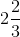3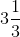— — — — — — — — — —

(d) Complete the table and find the solution to the equation m – 7 = 3.

 m 5 6 7 8 9 10 11 12 13 — — m – 7 — — — — — — — — — — —

Solutions:

(a)

 m 1 2 3 4 5 6 7 8 9 10 — — — m + 10 1 + 10 =11 2 + 10 =12 3 + 10 =13 4 + 10 =14 5 + 10 =15 6 + 10 =16 7 + 10 =17 8 + 10 =18 9 + 10 =19 10 = 10 =20 — — —
At m = 6 , m + 10 = 16So, m = 6 is the solution to the equation.

(b)

 t 3 4 5 6 7 8 9 10 11 — — — — — 5t 5 × 3 = 15 5 × 4 = 20 5 × 5 = 25 5 × 6 = 3 5 × 7 = 35 5 × 8 = 40 5 × 9 = 45 5 × 10 = 50 5 × 11 = 55 — — — — —

At t = 7 , 5t = 35

So, t = 7  is the solution to the equation.

(c)

 z 8 9 10 11 12 13 14 15 16 — — — — z / 3311 / 3 =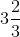12 / 3 = 4 13 / 3 =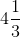14 / 3 =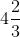15 / 3 = 5 16 / 3 =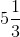— — — —

At z = 12 , z / 3 = 4

So, z = 12 is the solution to the equation

(d)

 m 5 6 7 8 9 10 11 12 13 — — m – 7 5 – 7 = -2 6 – 7 = -1 7 – 7 = 0 8 – 7 = 1 9 – 7 = 2 10 – 7 = 3 11 – 7 = 4 12 – 7 = 5 13 – 7 = 6 — —

At m = 10 , m – 7 = 3

So, m = 10 is the solution to the equation

## EX 11.5 QUESTION 5.

Solve the following riddles, you may yourself construct such riddles.

Who am I?(i) Go round a squareCounting every corner

Thrice and no more!

To get exactly thirty four!

(ii) For each day of the week

Make an upcount from me

If you make no mistake

You will get twenty three!(iii) I am a special number

Take away from me a six!

A whole cricket team

You will still be able to fix!

(iv) Tell me who I am

I shall give a pretty clue!

You will get me back

If you take me out of twenty two!

Solutions:

(I) I + 12 = 34 or x + 12 = 34

∴ By inspection,

22 + 12 = 34

So, I am 22.

(ii) Let I am ‘x’.

in a week there are 7 days

∴ up-counting from x for 7, the sum = 23

By inspections,

16 + 7 = 23

∴ x = 16

Thus I am 16.

(iii) Let the special number be x

and there are 11 players in the cricket team.

∴ Special Number -6 = 11

∴ x – 6 = 11

By inspection,

17 – 6 = 11

∴ x = 17

Thus I am 17.

(iv) Suppose I am ‘x’.

∴ 22 – I = I

or 22 – x = x

By inspection,

22 – 11 = 11

∴ x = 11

Thus I am 11.

error: Content is protected !!